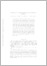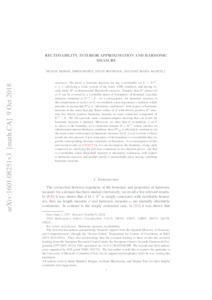# Rectifiability, interior approximation and Harmonic measure

Akman, Murat and Bortz, Simon and Hofmann, Steve and Martell, José María (2019) 'Rectifiability, interior approximation and Harmonic measure.' Arkiv för Matematik, 57 (1). pp. 1-22. ISSN 0004-2080Preview
Text
1601.08251v3.pdf - Submitted Version

We prove a structure theorem for any $n$-rectifiable set $E\subset\mathbb{R}^{n+1}, n \geq 1$, satisfying a weak version of the lower ADR condition, and having locally finite $\mathcal{H}^{n}$ ($n$-dimensional Hausdorff) measure. Namely, that $\mathcal{H}^{n}$-almost all of $E$ can be covered by a countable union of boundaries of bounded Lipschitz domains contained in $\mathbb{R}^{n+1}\setminus E$. As a consequence, for harmonic measure in the complement of such a set $E$, we establish a non-degeneracy condition which amounts to saying that $\mathcal{H}^{n}|_{E}$ is ''absolutely continuous'' with respect to harmonic measure in the sense that any Borel subset of $E$ with strictly positive $\mathcal{H}^{n}$ measure has strictly positive harmonic measure in some connected component of $\mathbb{R}^{n+1}\setminus E$. We also provide some counterexamples showing that our result for harmonic measure is optimal. Moreover, we show that if, in addition, a set $E$ as above is the boundary of a connected domain $\Omega\subset\mathbb{R}^{n+1}$ which satisfies an infinitesimal interior thickness condition, then $\mathcal{H}^{n}|_{\partial\Omega}$ is absolutely continuous (in the usual sense) with respect to harmonic measure for $\Omega$. Local versions of these results are also proved: if just some piece of the boundary is $n$-rectifiable then we get the corresponding absolute continuity on that piece. As a consequence of this and recent results in [AHM$^{3}$TV], we can decompose the boundary of any open connected set satisfying the previous conditions in two disjoint pieces: one that is $n$-rectifiable where Hausdorff measure is absolutely continuous with respect to harmonic measure and another purely $n$-unrectifiable piece having vanishing harmonic measure. [AHM$^{3}$TV] J. Azzam, S. Hofmann, J.M. Martell, S. Mayboroda, M. Mourgoglou, X. Tolsa and A. Volberg. Rectifiability of harmonic measure. arXiv:1509.06294, To appear in GAFA.View Item